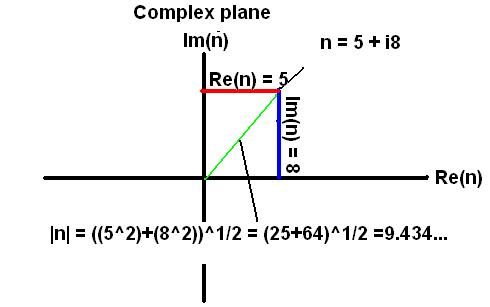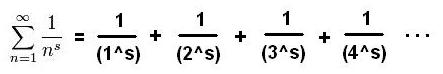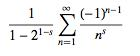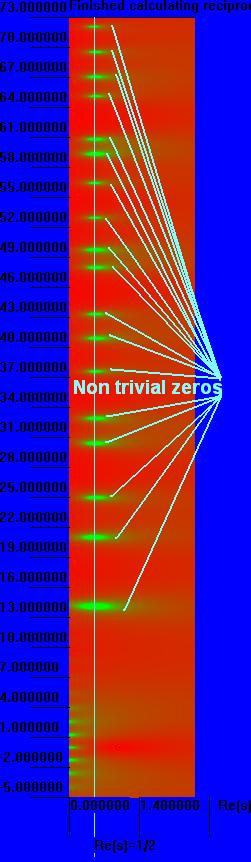What is the Riemann Hypothesis?      The simple explanation for non-math people will have to be very general and will miss out on a lot of what makes the Riemann hypothesis so interesting, but I'll put it here anyway because I seem to be getting a lot of hits for 'simple explanation'.      Basically there is an equation involving something called the Riemann zeta function, studied by a guy named Bernhard Riemann.  The Riemann hypothesis is based on an observation Riemann made about the equation:  Every input value of the equation that makes it go to zero seems to lie on the exact same line.  That might not sound very interesting but it is to mathematicians because these values keep coming up in the most crazy complicated places like quantum mechanics and number theory.  Most importantly the Riemann hypothesis is very closely related to prime numbers, something mathematicians don't understand very well.  The Riemann hypothesis is so famous because no one has been able to solve it for 150 years.  This is quite rare in math, because most theories can be proved or disproved fairly rapidly by someone with very bad hair.  I will try to give a more detailed description below, but if math scares you should probably stop reading while you still can...      First off I should explain what a complex number is, since the Riemann Zeta function works with them.  Many years ago mathematicians became bored with real numbers so they decided to make up some imaginary ones to make things more complicated.  You might think a complex number looks like two numbers but it is indeed one number.  A Complex number will be written in the form x + iy.  For example assume n is a complex number with the value 3 + i5.  The first part is called the real part (Re(n) = 3 in this example) and the part with the i is called the imaginary part (Im(n) = 5).  All normal numbers like 42 or 9.3 or 1 are really complex numbers in disguise we simply don't write the imaginary part because it is zero.  If we did they would look like 42 + i0 or 9.3 + i0 or 1 +i0.The Riemann Hypothesis states that all non trivial zeros of the Riemann zeta function have a real part equal to 0.5. But what does this mean?  A zero of a function is a value that you can put into the function and get zero to come out. For example if you have a function f(x) = x - 1, then x = 1 is a zero of this function because using it as x gives 1 - 1 = 0.  The Riemann Zeta function has some zeros that are easy to find which are of little interest but there are some other ones that are harder to find which is why the are called non-trivial.      Saying that the real part of any non-trivial zero is 0.5 means that when you put complex number values into the function that the real part of that value is always 0.5 if the number you get out of the function is zero.  Here is the Zeta function which is defined for Re(s) > 1.But wait a minute if the function is defined only for Re(s) > 1 then how can it have a real part that is 0.5?  Well it can't!  But thanks to the complicated magic spell called 'analytic continuation' we can turn this function into another function that has all the same values as the original but it has more values where the other function did not.  Here is the analytic continuation of the zeta function that is valid for Re(s) > 0.Here is a picture of the reciprocal absolute value of the analytic continuation of the zeta function.  The green part represents where it would be tending toward zero on the absolute value function.  These are the non-trivial zeros that people are always talking about, notice how they are all on the same line?.If you want to look at some of the interesting images generated by this function you can use this program.      The importance of the Riemann hypothesis is that a lot of questions about prime numbers can be reformulated into questions about the non trivial zeros of the Riemann zeta function.  This means that if you prove the Riemann hypothesis you prove a whole bunch of other conjectures too, because people have already made a proofs that have only one assumption: the Riemann hypothesis.